0
7635

# CAT Geometry Previous Year Questions PDF

Download important CAT Geometry Questions PDF based on previously asked questions in CAT exam. Practice geometry questions with solutions for CAT exam.

Take a free mock test for CAT

Question 1: Two circles with centres P and Q cut each other at two distinct points A and B. The circles have the same radii and neither P nor Q falls within the intersection of the circles. What is the smallest range that includes all possible values of the angle AQP in degrees?

a) Between 0 and 90

b) Between 0 and 30

c) Between 0 and 60

d) Between 0 and 75

e) Between 0 and 45

Question 2: In a triangle ABC, the lengths of the sides AB and AC equal 17.5 cm and 9 cm respectively. Let D be a point on the line segment BC such that AD is perpendicular to BC. If AD = 3 cm, then what is the radius (in cm) of the circle circumscribing the triangle ABC?

a) 17.05

b) 27.85

c) 22.45

d) 32.25

e) 26.25

Question 3: Consider obtuse-angled triangles with sides 8 cm, 15 cm and x cm. If x is an integer then how many such triangles exist?

a) 5

b) 21

c) 10

d) 15

e) 14

Question 4: Consider a square ABCD with midpoints E, F, G, H of AB, BC, CD and DA respectively. Let L denote the line passing through F and H. Consider points P and Q, on L and inside ABCD such that the angles APD and BQC both equal 120°. What is the ratio of the area of ABQCDP to the remaining area inside ABCD?

a) $\frac{4 \sqrt 2}{3}$

b) $2 + \sqrt{3}$

c) $\frac{10-3\sqrt{3}}{9}$

d) $1+\frac{1}{\sqrt3}$

e)  $2\sqrt{3}-1$

Question 5: Two circles, both of radii 1 cm, intersect such that the circumference of each one passes through the centre of the other. What is the area (in sq. cm.) of the intersecting region?

a) $\frac{\pi}{3}-\frac{\sqrt 3}{4}$

b) $\frac{2\pi}{3}+\frac{\sqrt 3}{2}$

c) $\frac{4\pi}{3}-\frac{\sqrt 3}{2}$

d) $\frac{4\pi}{3}+\frac{\sqrt 3}{2}$

e) $\frac{2\pi}{3}-\frac{\sqrt 3}{2}$

Question 6: Consider a right circular cone of base radius 4 cm and height 10 cm. A cylinder is to be placed inside the cone with one of the flat surfaces resting on the base of the cone. Find the largest possible total surface area (in sq. cm) of the cylinder.

a) $\frac{100\pi}{3}$

b) $\frac{80\pi}{3}$

c) $\frac{120\pi}{7}$

d) $\frac{130\pi}{9}$

e) $\frac{110\pi}{7}$

Question 7: Each side of a given polygon is parallel to either the X or the Y axis. A corner of such a polygon is said to be convex if the internal angle is 90° or concave if the internal angle is 270°. If the number of convex corners in such a polygon is 25, the number of concave corners must be

a) 20

b) 0

c) 21

d) 22

Question 8: There are two concentric circles such that the area of the outer circle is four times the area of the inner circle. Let A, B and C be three distinct points on the perimeter of the outer circle such that AB and AC are tangents to the inner circle. If the area of the outer circle is 12 square centimeters then the area (in square centimeters) of the triangle ABC would be

a) $\pi\sqrt{12}$

b) $9/\pi$

c) $9\sqrt3/\pi$

d) $6\sqrt3/\pi$

Question 9: Three horses are grazing within a semi-circular field. In the diagram given below, AB is the diameter of the semi-circular field with center at O. Horses are tied up at P, R and S such that PO and RO are the radii of semi-circles with centers at P and R respectively, and S is the center of the circle touching the two semi-circles with diameters AO and OB. The horses tied at P and R can graze within the respective semi-circles and the horse tied at S can graze within the circle centred at S. The percentage of the area of the semi-circle with diameter AB that cannot be grazed by the horses is nearest to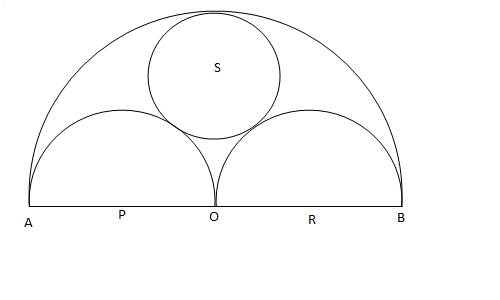a) 20

b) 28

c) 36

d) 40

Question 10: In the figure below, ABCDEF is a regular hexagon and $\angle{AOF}$ = 90° . FO is parallel to ED. What is the ratio of the area of the triangle AOF to that of the hexagon ABCDEF?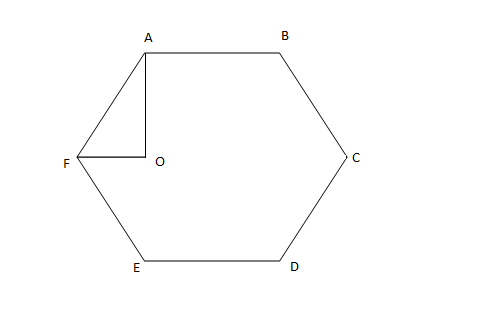a) 1/12

b) 1/6

c) 1/24

d) 1/18

Question 11: A vertical tower OP stands at the center O of a square ABCD. Let h and b denote the length OP and AB respectively. Suppose $\angle{APB}$ = 60° then the relationship between h and b can be expressed as

a) $2b^2 = h^2$

b) $2h^2 = b^2$

c) $3b^2 = 2h^2$

d) $3h^2 = 2b^2$

Question 12: In the triangle ABC, AB = 6, BC = 8 and AC = 10. A perpendicular dropped from B, meets the side AC at D. A circle of radius BD (with center B) is drawn. If the circle cuts AB and BC at P and Q respectively, the AP:QC is equal to

a) 1:1

b) 3:2

c) 4:1

d) 3:8

Question 13: In the diagram given below, $\angle{ABD}$ = $\angle{CDB}$ = $\angle{PQD}$ = 90° . If AB:CD = 3:1, the ratio of CD: PQ is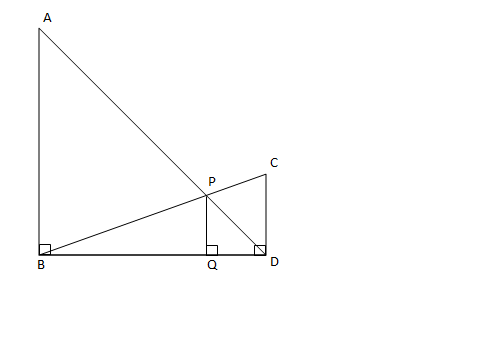a) 1 : 0.69

b) 1 : 0.75

c) 1 : 0.72

d) None of the above.

Question 14: In the figure below, AB is the chord of a circle with center O. AB is extended to C such that BC = OB. The straight line CO is produced to meet the circle at D. If $\angle{ACD}$ = y degrees and $\angle{AOD}$ = x degrees such that x = ky, then the value of k is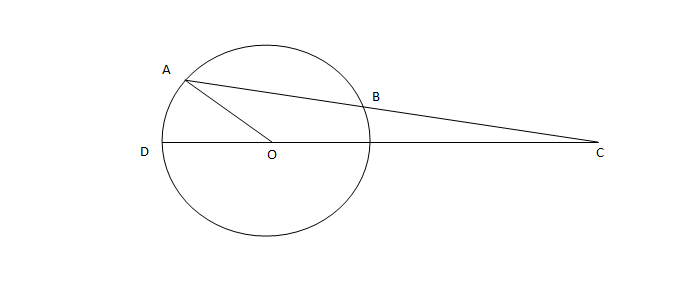a) 3

b) 2

c) 1

d) None of the above.

Question 15: In the figure below, the rectangle at the corner measures 10 cm × 20 cm. The corner A of the rectangle is also a point on the circumference of the circle. What is the radius of the circle in cm?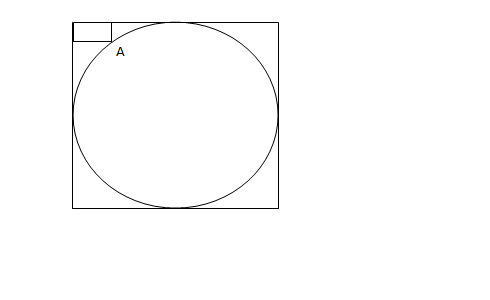a) 10 cm

b) 40 cm

c) 50 cm

d) None of the above.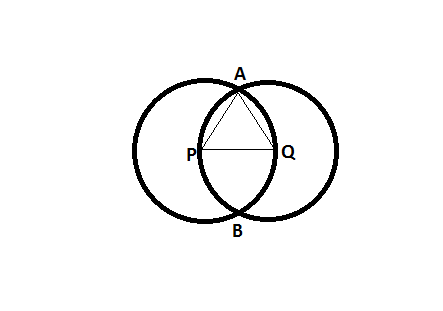To know the range, we have to take the limiting case.

The limiting case in this case is when the circles pass through each other’s centers.

In this case, PQ = AP = AQ => They form an equilateral triangle => angle AQP = 60 degrees.

So, the maximum possible angle is 60 degrees.

Another limiting case is when the circles touch each other externally.

In this case, angle AQP = 0 degrees.

Hence, the range is 0 to 60.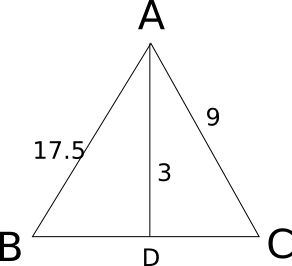Let x be the value of third side of the triangle. Now we know that Area = 17.5*9*x/(4*R), where R is circumradius.

Also Area = 0.5*x*3 .

Equating both, we have 3 = 17.5*9 / (2*R)

=> R = 26.25.

For obtuse-angles triangle, $c^2 > a^2 + b^2$ and c < a+b
If 15 is the greatest side, 8+x > 15 => x > 7 and $225 > 64 + x^2$ => $x^2$ < 161 => x <= 12
So, x = 8, 9, 10, 11, 12
If x is the greatest side, then 8 + 15 > x => x < 23
$x^2 > 225 + 64 = 289$ => x > 17
So, x = 18, 19, 20, 21, 22
So, the number of possibilities is 10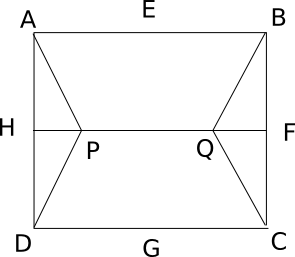Consider side of square as 10 units. So HD=5 and HP=$\frac{5}{\sqrt3}$ . So now area of triangle HPD=$\frac{12.5}{\sqrt3}$. Also Area APD=Area BQC=2*AreaHPD=$\frac{25}{\sqrt3}$. So numerator of required answer is $100-\frac{50}{\sqrt3}$ and denominator as $\frac{50}{\sqrt3}$. Solving we get answer as $2\sqrt{3}-1$.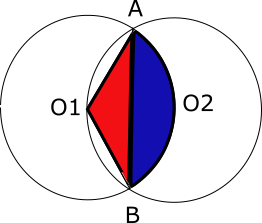The circumferences of the two circle pass through each other’s centers. Hence, O1A = O1B=O1O2 = 1cm

By symmetry, the line joining the two centres would be bisect AB and would be bisected by AB. As the line joining the center to the midpoint of a chord is perpendicular to the chord, O1O2 and AB are perpendicular bisectors of each other. Suppose they intersect at point P. Hence, O1P = 1/2 cm. Hence, angle O1AB = sin x = (1/2)/1 = 1/2. Hence, O1AB = 30°. By symmetry, O1BA = 30° and hence angle at centre O1 = 120°.

In the above, the required area is 2 times A(segment ABO2)(blue region). And A(segment ABO2)(blue region) = A(sector O2AO1B)(blue + red) – A(triangleO1AB )(red)

Area of sector = 120°/360° * $\pi * 1^2$ = $\pi/3$

Area of triangle = 1/2 * b * h = 1/2 * (2* 1 cos 30°) * (1/2) = √3/4

Hence, required area = $\frac{\pi}{3}-\frac{\sqrt 3}{4}$ . Hence so the required area is 2 times the above value which is $\frac{2\pi}{3}-\frac{\sqrt 3}{2}$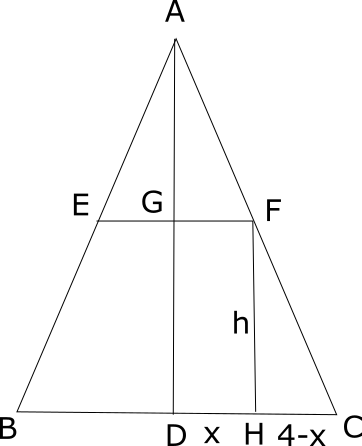Trangle AGF and triangle FHC are similar.
So, $\frac{10-h}{x}=\frac{h}{4-x}$
=> $\frac{10-h}{h}=\frac{x}{4-x}$
=> $\frac{10}{h}-1=\frac{x}{4-x}$
=> $\frac{10}{h}=\frac{4}{4-x}$
=> $h=\frac{10}{4}(4-x)$

Total surface area= $2 \pi x^2 + 2 \pi x h= 2 \pi (x^2 + x \frac{10}{4}(4-x)=2 \pi (10x – \frac{3}{2} (x^2)=2 \pi (-\frac{3}{2}(x-\frac{10}{3}^{2})+ \frac{50}{3})$

This value will be maximum when the negative factor is minimum i.e. 0 because it is multiplied by a square. Thus, when x=10/3 the surface area is max.

So, the surface area is $\frac{100}{3} \pi$

Let the total number of sides be x.

(25*90)+(x-25)*270 = (x-2)180

x = 46

Number concave corners = x-25 = 46-25 = 21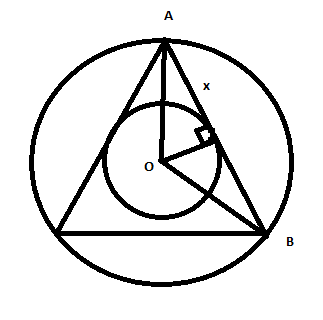Let R ,r be radius of big and small circles respectively. We know that R=2r.

And since area = 12 ;

$R^2 = \frac{12}{\pi}$.

By pythagoras theorem in the small triangle with side ‘x’ we have x = 3 / $\sqrt{\pi}$.

This triangle is a 30-60-90 right triangle. => Triangle ABC is an equilateral triangle.

So side of triangle ABC = 2x = 6 / $\sqrt{\pi}$.

Also Angle OAB = 30 (as side opposite to the angle is half of the hypotenuse ) .

Hence Angle CAB = 60. Hence required triangle is an equilateral triangle. So area = $\sqrt{3}$ * (2x)$^2$ / 4 . Substituting we get . Area = $9\sqrt3/\pi$.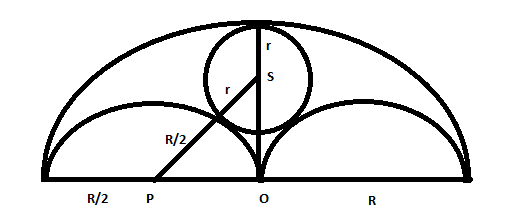Let R be radius of big circle and r be radius of circle with centre S. Radius of 2 semicircles is R/2.

From Right angled triangle OPS, using pythagoras theorem we get

$(r+0.5R)^2 = (0.5R)^2 + (R-r)^2$ . We get R=3r .

Now the area of big semicircle that cannot be grazed is Area of big S.C – area of 2 semicircle – area of small circle. We get equal to 5*$\pi*R^2$/36  this is about 28 % of the area $\pi*R^2$/2 . Hence option B.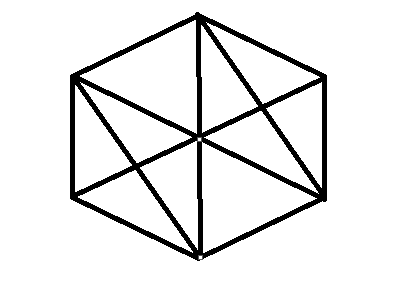When the hexagon is divided into number of similar triangle AOF we get 12 such triangles . Hence required ratio of area is 1/12.

Consider the triangle APB.  $\angle$P = 60 and AP = BP => APB is an equilateral triangle.

Hence AP = $b$

AO = $\sqrt{\dfrac{b^2}{4} + \dfrac{b^2}{4}}$ = $\dfrac{b}{\sqrt{2}}$

$\text{AO}^2 + \text{OP}^2 = \text{AP}^2$

$\dfrac{b^2}{2} + h^2 = b^2$

$2h^2 = b^2$

Hence, option B is the correct answer.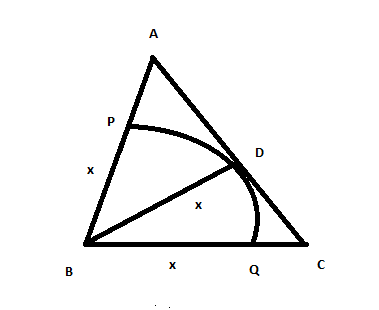Let BD = x .Semi-perimeter of triangle ABC = 12. Now by herons formula area of ABC is 24. Also Area = 0.5*x*10 . We get x = 24/5 .  AP = 6/5  and CQ = 16/5 . Hence the required ratio is 3:8.

Let BQ = z , QD = y , PQ = x.

From similar triangles PQD and ABD we have

(y/x) = (z+y)/3 .

Also from  similar triangles PQB and CBD we have

(z/x) = z+y .

Solving we get z = 3*y.

Now required ratio is (z+y)/z.

We get eual to 4/3 which is equal to 1:0.75.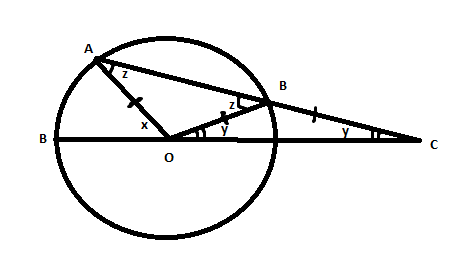Since Angle BOC = Angle BCO = y.

Angle OBC = 180-2y .

Hence Angle ABO =  z = 2y = Angle OAB.

Now since x is exterior angle of triangle AOC .

We have x = z + y = 3y.

Hence option A.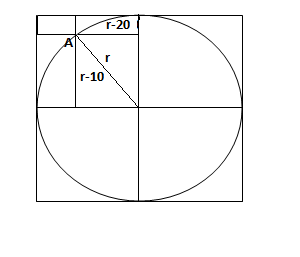Using pythagoras we have $r^2 = (r-10)^2 + (r-20)^2$.